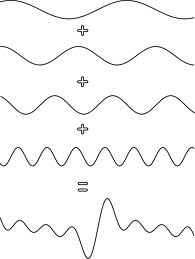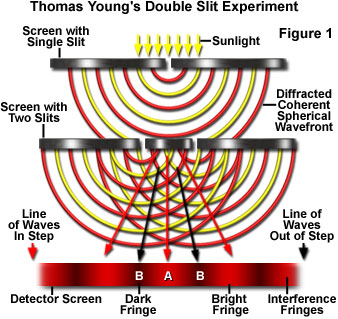## 2008-03-25

### Analysing Interference of Waves

Principle of Superposition

1. The principle of superposition states that at any instant or moment, the wave displacement of the combined motion of any number of interacting waves at a point is the sum of the displacements off all the component waves at that point.

2. a + a = 2a

a + -a = 0

-a + -a = -2aSource: www.pitt.edu

Interference of Waves

1. Interference is the superposition of two waves originating from two coherent sources. Sources which are coherent produce waves of the same frequency,f, amplitude,a, and are in phase.

2. The superposition of two waves emitted from coherent sources gives either constructive or destructive interference.

3. Constructive interference occurs when the crests or throughs of both waves coincide to produce a wave with crests and troughs of maximum amplitude.4. Destructive interference occurs when the crest of one wave coincides with the trough of the other wave, thus cancelling each other with the result that the resultant amplitude is zero.

5. An antinode is a point where a constructive interference occurs, whereas a node is a point where destructive interference occurs. The antinodal lines join all antinodes and the nodal line joins all nodes.

Relationship between lambda, a, x and D (will be discussed later)

Interference of Light waves

1. Waves emitted from two coherent sources have the same frequency,f or wavelength and in phase.2. Light emitted by a single source of consists of waves which extend over a wide range of wavelengths and are not in phase. because of this, it is difficult to have two sources of light which are coherent.

3. In 1801, Thomas Young produced two coherent light sources in his experiment now referred to as Young's double slit experiment.

a) Yellow light emitted by a sodium-vapour lamp has a very narrow frequency band. for all its practical purposes, it can be considered as a monochromatic light which is light of only one frequency or wavelength.

b) Slits s1 and s2 give rise to two coherent light sources since the light passing through them are from the same monochromatic light, the sodium vapour light.

c) Interference occurs as a result of the superposition of the two light waves originating from s1 and s2. A pattern consisting of a series of parallel and alternating bright and dark fringes is formed.

d) The bright fringes are the region where constructive interference occurs, whereas the dark fringes are regions of destructive interference.

#### 2 comments:Anonymous said...

this is quite sufficient but is it possible to post some experiment or picturesAnonymous said...

the formula must be included coz its in the spm syllubus